# Rajasthan Board Class 10 Maths Important Questions And Answers

Rajasthan Board Class 10 students find the Maths subject to be difficult. It is even seen that many of them just for the sake of scoring well, mug up the formulas and at times even the steps of solving the questions. However, if a student is not thorough with the concepts, they will not be able to ace the Maths subject. Hence, for the convenience of the students here, we have provided access to Rajasthan Board Class 10 Maths Important Questions. Solving these important questions will help RBSE Class 10 students to brush up their knowledge about Maths. They might be able to assess the difficulty level of the questions asked. One of the best ways to get acquainted with answering different questions of various difficulty level is to refer to the RBSE Class 10 Maths Important Questions And Solutions.

## RBSE Class 10 Maths Important Questions With Solutions

Practising these Rajasthan Board Class 10 Maths Important Questions with Solutions will help the students to save a lot of time while writing the exams. At the same time, they will also be able to devise a better exam strategy. Students can download the pdf and use it to sharpen their understanding of the various concepts covered in Class 10 Maths of Rajasthan Board.

### Rajasthan Board Class 10 Maths Important Questions And Answers

Question 1: Write the number of circles passing through three non-collinear points.

Solution:
If the circle needs to pass through all the three non-collinear points, only one circle can be drawn.

In the figure, P, Q, R are three non- collinear points.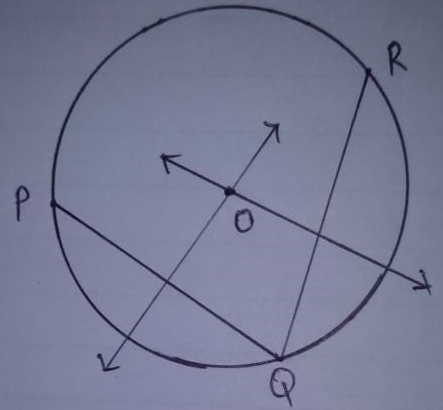Question 2: Find the probability of getting a prime number on throwing the die once.

Solution:
Total outcomes = 6 = {1, 2, 3, 4, 5, 6}

Sample space = S = {2, 3, 5}

The total number of prime numbers that can occur on the dice is 3.

P (getting a prime number) = Number of prime number outcomes / Total number of outcomes

= 3 / 6

= 1 / 2

Question 3: What is the shape of red signal light in traffic signs?

Solution:
The signal lights are in the shape of a circle.

Question 4: Solve: 1 / (x – 3) + 1 / (x – 7) = 1 / (x – 1) + 1 / (x – 9).

Solution:
1 / [x – 3] + 1 / [x – 7] = 1 / [x – 1] + 1 / [x – 9] [x – 7 + x – 3] / [x – 3] [x – 7]

= [x – 9 + x – 1] / [x – 1] [x – 9] [2x – 10] / [x2 – 10x + 21]

= [2x – 10] / [x2 – 10x + 9] (2x – 10) (x2 – 10x + 9)

= (2x – 10) (x2 – 10x + 21) (2x – 10) (x2 – 10x) + (2x – 10) 9

= (2x – 10) (x2 – 10x) + (2x – 10) 21 18x – 90

= 42x – 210

24x = 120

x = 5

Question 5: Write the sum of powers of prime factors of 196.

Solution:
196 is a composite number.

Prime factorization:

196 = 2 × 2 × 7 × 7 196

= 2² × 7²

The sum of the exponents in the prime factorization is 2 + 2 = 4.

Question 6: Write the value of cos 50o cosec 40o.

Solution:
cos 50o × cosec 40o

= cos 50o × cosec (90o – 50o)

= cos 50o × sec 50o

= cos 50o × [1 / (cos 50o)]

= 1

Question 7: Write the locus of the point equidistant from the two given points.

Solution: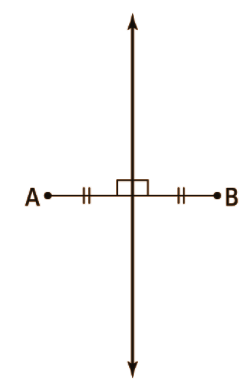The locus of points equidistant from two given points is the perpendicular bisector of the segment that joins the two points.

Question 8: HCM and LCM of two integers are 12 and 336, respectively. If one integer is 48, then find the other integer.

Solution:
HCF and LCM of two integers are 12 and 336. One of the integers is 48.

First number × Second number = HCF × LCM

Let the other integer be a.

48 * a = 12 * 336

a = [12 * 336] / 48

a = 4032 / 48

a = 84

Question 9: If the ratio of corresponding medians of two similar triangles is 9: 16, then find the ratio of their areas.

Solution:
The theorem states that “the ratio of the areas of two triangles is equal to the square of the ratio of their corresponding medians”.

Thus, we have,

Ratio of medians = 9:16 = 9 / 16

Ratios of their areas = [9 / 16]2

= 81 / 256

Question 10: Express the trigonometric ratio of tan A in terms of sec A.

Solution:
tan2 A = sec2 A – 1

tan A = √sec2 A – 1

Question 11: In the given figure, O is the centre of a circle and two tangents QP and QR are drawn on the circle from a point Q lying outside the circle. Find the value of angle POR.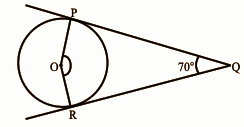Solution:
∠OPQ = 90o

∠ORQ = 90o

The sum of the angles of the quadrilateral OPQR is 360o.

∠OPQ + ∠ORQ + ∠PQR + ∠POR = 360o

90o + 90o + 70o + ∠POR = 360o

∠POR = 360o – 250o

∠POR = 110o

Question 12: Find the circumference of a circle whose diameter is 14cm.

Solution:
The radius will be half of the diameter.

The diameter of the circle = 14 cm

Radius of the circle = 14 / 2 = 7 cm

Circumference = 2Πr

= 2 * Π * 7

= 2 * (22 / 7) * 7

= 43.98 cm

Question 13: Find the area of the shaded portion in the given figure: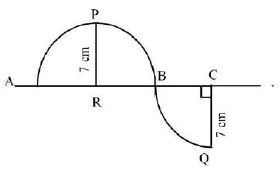Solution:
Area of the shaded portion = (1 / 2) 𝛑r2 + (1 / 4) 𝛑r2

= (3 / 4) 𝛑r2

= (3 / 4) * (22 / 7) * (72)

= 115.5 cm2

Question 14: A CCTV camera is placed on the top of a straight 12-meter high pole in such a way that traffic can be seen beyond 13 meters of the line of sight of it. Find the distance from the foot of the pole beyond which the traffic is visible.

Solution:
Height of the pole = 12m

Length of the line of sight = 13m

Hypotenuse2 = Base2 + Perpendicular2

Base2 = Hypotenuse2 – Perpendicular2

= 132 – 122

= 169 – 144

= 25

Base2 = 25

Base = 5m

Question 15: Find the square root of 6889 by using the Dwandwa Yoga Method.

Solution:
Number = 6889

83 | 6889

83 | 83

| 1

6889 = 83 * 83 6889 = 832

√6889 = √832

√6889 = 83

Question 16: By using the division algorithm method find the quotient and remainder when polynomial P (x) = x4 – 3x2 + 4x – 3 is divided by g (x) = x2 + (1 – x).

Solution: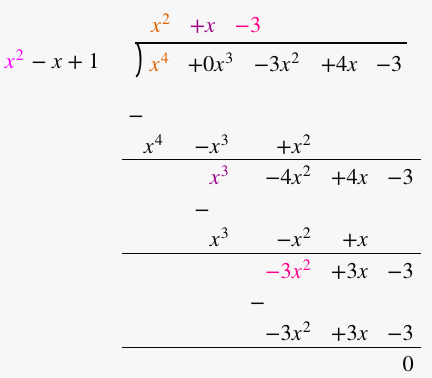Quotient: x2 + x – 3 Remainder: 0

Question 17: Name the type of quadrilateral formed by the points (4, 5), (7, 6), (4, 3), (1, 2).

Solution:
Let the points (4, 5), (7, 6), (4, 3), and (1, 2) be representing the vertices A, B, C, and D of the given quadrilateral, respectively.

AB = √(4 – 7)2 + (5 – 6)2

= √(-3)2 + (-1)2

= √9 + 1

= √10

BC = √(7 – 4)2 + (6 – 3)2

= √(3)2 + (3)2

= √9 + 9

= √18

CD = √(4 – 1)2 + (3 – 2)2

= √(3)2 + (1)2

= √9 + 1

= √10

AD = √(4 – 1)2 + (5 – 2)2

= √(3)2 + (3)2

= √9 + 9

= √18

Diagonal AC = √(4 – 4)2 + (5 – 3)2

= √(0)2 + (2)2

= √0 + 4

= 2

Diagonal CD = √(7 – 1)2 + (6 – 2)2

= √(6)2 + (4)2

= √36 + 16

= √52

The opposite sides of this quadrilateral are of the same length.

However, the diagonals are of different lengths.

Therefore, the given points are the vertices of a parallelogram.

Question 18: A vessel is in the form of a hollow hemisphere. The diameter of the hemisphere is 14 cm. Find the inner surface area of the vessel.

Solution:
D = 14cm (given)

R = 14 / 2

= 7cm

CSA of hemisphere = 2πr2

= 2 × [22 / 7] × 7 × 7

= 44 × 7

= 308 cm2

Question 19: A car travels 260 km distance from a place A to place B, at a uniform speed, 65 km/hr passes through all thirteen green traffic signals, 4 minutes at the first signal, 7 minutes at the second signal, 10 minutes at a third signal and so on stops for 40 minutes at the thirteenth signal. How much total time does it take to reach place B? Solve by suitable Mathematical Method.

Solution:
Time sequence forms an A.P: 4, 7, 10…… a = 4 d = 7 − 4 = 3

For the 13th station, time = 40

Total time is the sum of the first thirteen terms of the sequence

S = [n / 2] {2a + (n − 1) d}

S = [13 / 2] {2(4) + (13 − 1) 3}

S = [13 / 2] [8 + 36]

S = [13 / 2] × 44 S = 286

S = [286 / 60] hour

= 4 + [286 / 60]

= [240 + 286] / 60

= 526 / 60

= 8.76 hours

Question 20: Per day expenses of 25 families of the frequency distribution of a Dhani of a village is given as follows:

 Per day expense (in Rs) 25 – 35 35 – 45 45 – 55 55 – 65 65 – 75 Number of families 3 7 6 6 3

Find the mean expense of families by Direct Method.

Solution:

 Per day expense (in Rs) 25 – 35 35 – 45 45 – 55 55 – 65 65 – 75 Number of families 3 7 6 6 3 Midpoint 30 40 50 60 70 fixi 90 280 300 360 210

Mean = ∑fi xi / ∑fi
= 1240 / 25 = 49.6

Question 21: Find the value of (tan 67°) / (cot 23°).

Solution:
(tan 67°) / (cot 23°)

tan θ = cot (90o – θ)

tan 670 = cot (90o – 67o)

= cot 23o

= cot 23o / cot 23o

= 1

Question 22: In the figure, ΔOPR ~ ΔOSK , ∠POS = 125˚ and ∠PRO = 70˚. Find the values of ∠OKS and ∠ROP.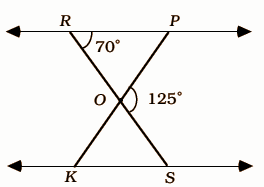Solution:
Given that, Δ OPR ~ Δ OSK, ∠POS = 125˚ and ∠PRO = 70˚ Sum of the supplementary angles = 180o

∠POS + ∠POR = 180o

125o + ∠ROP = 180o

∠ROP = 180o – 125o

∠ROP = 55o

∠OKS = ∠PRO [Since triangle Δ OPR ~ Δ OSK]

∠OKS = 70o

Question 23: Find quadratic polynomials whose sum and product of zeros are 8 and 12 respectively.

Solution:
A quadratic polynomial when the sum and products are given by f (x) = k, where k is constant.

Sum = 8

Product = 12

Polynomial = x2 – (sum of zeroes) x + product of zeroes = x2 – 8x + 12

Question 24: The angle of elevation of the top of the tower from two points at a distance of a and b from the base of the tower in the same straight line with it are complementary. Prove the height of the tower is √ab.

Solution: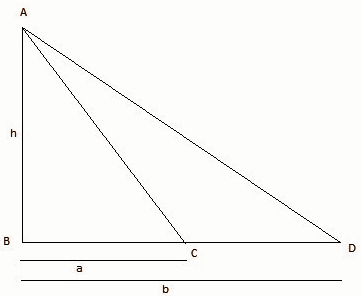Height of the tower = h

Distance between the tower and point one = BC = A meter

Distance between the tower and point one = BD = B meter

The ∠ACB + ∠ADB = 90° (complementary angle) and

∠ABC = ∠ABD = 90°

∠ACB = θ

In right-angle triangle ABC tan θ = AB / BC — (1)

tan θ = h / a

In right-angle triangle ABD tan (90 – θ) = AB / BD — (2)

tan (90 – θ) = h / b

Now multiplying equation 1 with equation 2,

tan θ * cot θ = (h / a) * (h / b)

tan θ * (1 / tan θ)= h2 / ab

1 = h2 / ab

h= ab

h = √ab

Question 25: Circumference of a circle is equal to the perimeter of a square if the area of a square is 484 sq. meter, then find the area of the circle.

Solution:
If the side of square = xm, then perimeter of square 4 × x Area of square = x2

Area of square = 484 sq m2

x2 = 484 x = √484 x = 22 m

Perimeter of square = 4x = 4 × 22 = 88 m

Circumference of circle = Perimeter of square

⇒ 2πr = 88

⇒ 2 × (22 / 7) × r = 88

r = [88 × 7] / [2 × 22]

= 14 m

Area of the circle = πr2

= (22 / 7) × 14 × 14

= 616 sq m.

Thus, the area of the circle = 616 sq m.

Question 26: A card is drawn from a well-shuffled pack of 52 cards. Find the probability of the following that the card is:
(a) Black
(b) Ace of Heart

Solution:
(a) Out of 52 cards, 26 cards are black.

Favourable number of outcomes = 26

P (black card)

= 26 / 52

= 1 / 2

(b) There are 4 aces out of which one is a heart.

Favourable number of outcomes = 1

P (ace of heart) = 1 / 52

(c) Out of 52 cards, there are 13 cards of spades

Favourable number of outcomes = 13

P (spade) = 13 / 52

= 1 / 4

Question 27: If the second and third terms of an Arithmetic Progression are 3 and 5 respectively, then find the sum of the first 20 terms of it.

Solution:
a2 = 3

a3 = 5

a3 – a2 = 5 – 3 = 2 [common difference]

a2 – d = a1

3 – 2 = a1

a1 = 1

Sn = (n / 2) (2a + [n – 1] d)

S20 = (20 / 2) (2 * 1 + 19 * 2)

= 10 [2 + 38]

= 10 

= 400

Question 28: Prove that the angle between the two tangents drawn from an external point to a circle is supplementary to the angle subtended by the line-segment joining the points of contact at the centre.

Solution: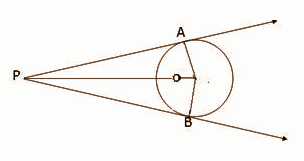Consider a circle with centre O. Let P be an external point from which two tangents PA and PB are drawn to the circle which are touching the circle at point A and B respectively and AB is the line segment, joining the point of contacts A and B together such that it subtends ∠AOB at centre O of the circle.

It can be observed that OA ⊥ PA ∴ ∠OAP = 90°

Similarly, OB ⊥ PB ∴ ∠OBP = 90°

Sum of all interior angles = 360º

∠OAP + ∠APB + ∠PBO + ∠BOA = 360º

⇒ 90º + ∠APB + 90º + ∠BOA = 360º

⇒ ∠APB + ∠BOA = 180º

∴ The angle between the two tangents drawn from an external point to a circle is supplementary to the angle subtended by the line-segment joining the points of contact at the centre.

Question 29: Prove that:
[i] √[1 + cos θ] / [1 – cos θ] = cosec θ + cot θ
[ii] tan θ / [1 – cot θ] + cot θ / [1 – tan θ] = 1 + tan θ + cot θ

Solution:
[i] √[1 + cos θ] / [1 – cos θ]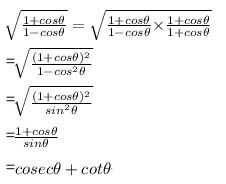[ii] tan θ / [1 – cot θ] + cot θ / [1 – tan θ] = 1 + tan θ + cot θ

LHS = tanθ / (1 – cotθ) + cotθ / (1 – tanθ)

= tanθ / (1 – 1 / tanθ) + (1 / tanθ) / (1 – tanθ)

= tan²θ / (tanθ – 1) + 1 / tanθ (1 – tanθ)

= tan³θ / (tanθ – 1) – 1 / tanθ (tanθ – 1)

= (tan³θ – 1) / tanθ (tanθ – 1)

= (tanθ – 1)(tan²θ + 1 + tanθ) / tanθ (tanθ – 1)

= (tan²θ + 1 + tanθ) / tanθ

= tanθ + cotθ + 1

Question 30: A well of diameter 7 m is dug and earth from digging is evenly spread out to form a platform 22 m × 14 m × 2.5 m. Find the depth of the well.

Solution:
The diameter of the well = 7 m

The radius of the well = 7 / 2 m

L * B * H = πr2h

22 * 14 * 2.5 = 22 / 7 × 7 / 2 × 7 / 2 × h

770 / 38.485 = h

h = 20cm

Question 31: Prove the √6 is an irrational number.

Solution:
Let √6 be a rational number, then √6 = p ÷ q , where p,q are integers , q not = 0 and p,q have no common factors ( except 1 )

=> 6 = p² ÷ q² => p² = 2q² …………….(i)

As 2 divides 6q², so 2 divides p² but 2 is a prime number

=> 2 divides p

Let p = 2m, where m is an integer.

Substituting this value of p in (i),

(2m)² = 6q² => 2m² = 3q²

As 2 divides 2m², 2 divides 3q²

=> 2 divides 3 or 2 divides q²

But 2 does not divide 3, therefore, 2 divides q²

=> 2 divides q

Thus, p and q have a common factor 2.

This contradicts that p and q have no common factors (except 1).

Hence, the supposition is wrong. Therefore, √6 is an irrational number.

Question 32: A box contains 7 red marbles, 10 white marbles and 5 green marbles. One marble is taken out of the box at random. What is the probability that the marble taken out will be
(i) not red?
(ii) white?
(iii) green?

Solution:
Number of red marbles = 7

Number of white marbles = 10

Number of green marbles = 5

Total marbles = 7 + 10 + 5 = 22

Probability of getting not red marble = 15 / 22

Probability of getting white marble = 10 / 22​

Probability of getting green marble = 5 / 22

Question 33: Seven spheres of equal radii are made by melting a silver-cuboid of dimensions 8cm × 9cm ×11cm. Find the radius of a silver sphere.

Solution:
The total volume before melting equals the total volume after melting.

The volume of the cuboid is equal to the volume of seven spheres. Vc = 7Vs
​abc = 7 × [4 / 3] × πr3

8 × 9 × 11 ≈ 7 × [4 / 3] × [22 / 7] × r3

792 = 29.321r3

r3 = 792 / 29.321

r3 = 27

r = 3 cm

Question 34: (i) If the distance between the points (x, 3) and (5, 7) is 5, then find the value of x.
(ii) Find the ratio in which the line 3x + y = 9 divides the line segment joining the points (1, 3) and (2, 7).

Solution:
[i] Let (x1, y1) = (x, 3)

(x2, y2) = (5, 7)

d = √(x2 – x1)2 + (y2 – y1)2

5 = √(5 – x)2 + (7 – 3)2

d = 5

On squaring of both sides, 25 = (5 – x)2 + 16 25 – 16 = (5 – x)2

9 = (5 – x)2

(5 – x) = ± 3

If x = 3,

5 – x = 3

5 – 3 = x

x = 2

If x = -3,

5 – (-3) = 3

x = 8

x = 2, 8

[ii] Let the line divides the points in k:1 ratio according to section formula (2k + 1 / k + 1, 7k + 3 / k + 1) = (x, y)

It must satisfy the given equation so

3(2k + 1 / k + 1) + (7k + 3 / k + 1) = 9

6k + 3 + 7k + 3 / k + 1 = 9

13k + 6 = 9k + 9

13k – 9k = 9 – 6

4k = 3

k = 3 / 4

Hence, the ratio is 3:4.

Question 35: ABC is a right-angled triangle whose ∠B is a right angle. If points D and E are situated on the sides AB and BC respectively, then prove that AE2 + CD2 = AC2 + DE2.

Solution: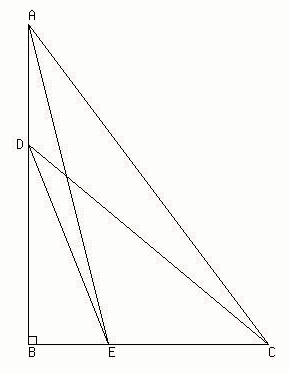ΔABE is a right triangle, right-angled at B AB² + BE² = AE²……………..(1) (by the Pythagoras theorem)

ΔDBC is a right triangle, right-angled at B

DB² + BC² = CD²…………….(2) (by the Pythagoras theorem)

AE² + CD² = (AB² + BE²) + (BD² + BC²)

AE² + CD² = (AB² + BC²) + (BE² + BD²)……(3) [Rearranging the terms]

ΔABC is a right triangle, AB² + BC² = AC²…………….(4) (by the Pythagoras theorem)

ΔDBE is a right triangle DB² + BE² = DE²………………(5) (by the Pythagoras theorem)

AE² + CD² = (AB² + BC²) + (BE² + BD²)

AE² + CD² = AC² + DE²

Question 36: The diagonal of a rectangular field is 25 meters more than the shorter side. If the longer side is 23 meters more than the shorter side, find the sides of the field.

Solution:
Let the shorter side of the rectangular field be ‘x’ meters.

Therefore the longer side will be (x + 23) meters and the length of the diagonal will be (x + 25) meters.

The diagonal divides the rectangular into two right-angled triangles and the diagonal is the common side of the two triangles and it is also the longest side of the triangles i.e. the hypotenuse.

By Pythagoras Theorem, (Diagonal)² = (Smaller Side)² + (Longer Side)²

(x + 25)² = (x)² + (x + 23)²

x² + 50x + 625 = x² + x² + 46x + 529

x² + 50x – 46x + 625 – 529 = 2x2

x² + 4x + 96 = 2x2

x2 – 4x – 96 = 0

x2 – 12x + 8x – 96 = 0

x(x – 12) + 8(x – 12) = 0

x = 12, -8

x = 12m as length cannot be possible.

So the length of the shorter side is 12 meters and the length of the longer side is 12 + 23 = 35 meters.

Question 37: Prove that (tan A – sin A) / (tan A + sin A) = (sec A – 1) / (sec A + 1).

Solution:
LHS = [tan A – sin A] / [tan A + sin A]

= [sin A / cos A] – sin A / [sin A / cos A] + sin A

= sin A [(1 / cos A) – 1] / sin A [(1 / cos A) + 1]

= [sec A – 1] / [sec A + 1]

Question 38: PQRS is a trapezium in which PQ || RS and its diagonals intersect each at the point O. Prove that PO / QO = RO / SO.
Solution:
As PQ || RS and PR and QS are transversals,

∠OSR = ∠OQP [alternate angles]

∠ORS = ∠OPQ [alternate angles]

△SOR ~ △POQ

SO / PO = RO / OQ = SR / PQ

SO / PO = RO / QO

QO / PO = RO / SO [by alterendo]

Question 39: Solve the following pair of linear equations by graphical method: 2x + y = 6, 2x – y = 2. Thus find the value of p in the relation 6x + 7y = p.

Solution:
2x + y = 6 —- (1)

2x – y = 2 —- (2)

4x = 8 x = 2

Put x = 1 in (1),

2 * 2 + y = 6

y = 6 – 4

y = 2

In 6x + 7y = p

6 * 2 + 7 * 2

= 12 + 14

= 26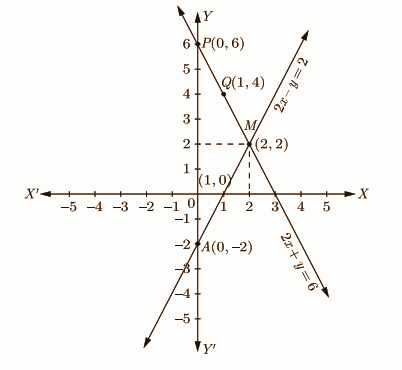Question 40: The cost of 5 apples and 3 oranges is Rs. 35 and the cost of 2 apples and 4 oranges is Rs. 28. Formulate the problem algebraically and solve it graphically.

Solution:
Let the cost of 1 apple be x and the cost of 1 orange be y.

According to question

5x + 3y = 35 – – – – – (i)

2x + 4y = 28 – – – – (ii)

Multiply by 2 in equation (i) and by 5 in equation (ii)

Now, => 10x + 6y = 70 – – – – (i)

=> 10x + 20y = 140 – – – (ii)

By subtraction equation (i) from (ii)

=> 14y = 70

=> y = 10 / 14

=> y = 5

Putting the value of y in equation (i)

5x + (3 * 5) = 35

=> 5x = 35 – 15

=> x = 20 / 5

=> x = 4

Hence, the cost of an apple = ₹4 and the cost of an orange = ₹5.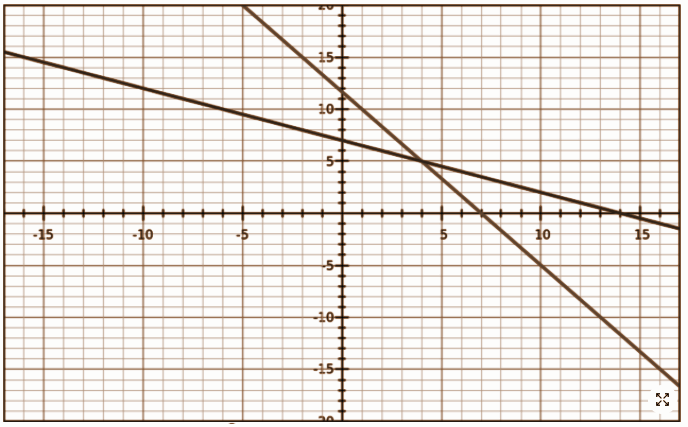Meanwhile, find also some extra RBSE Class 10 Maths Important Questions for the students to solve for practise.

Q.1 Two players Ram and Shyam plays a chess match. It is given that probability of winning of the match by Ram is 4/5th.  Find the probability of winning of the match by Shyam.

Q. 2  Find a quadratic equation whose sum of zeros and product are -3 and 2 respectively.

Q. 3 If the ratio of the corresponding sides of two triangles is equal, then to prove that the triangles are similar.